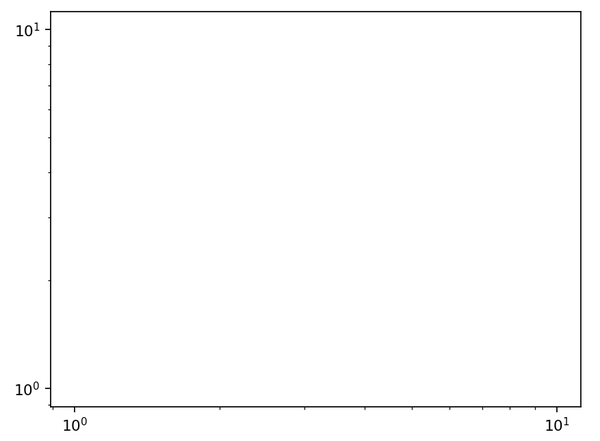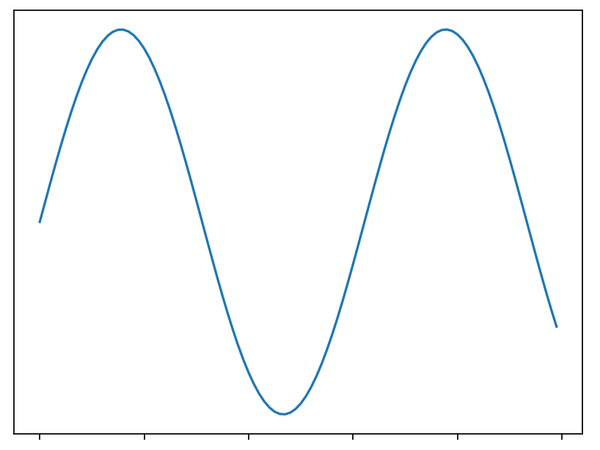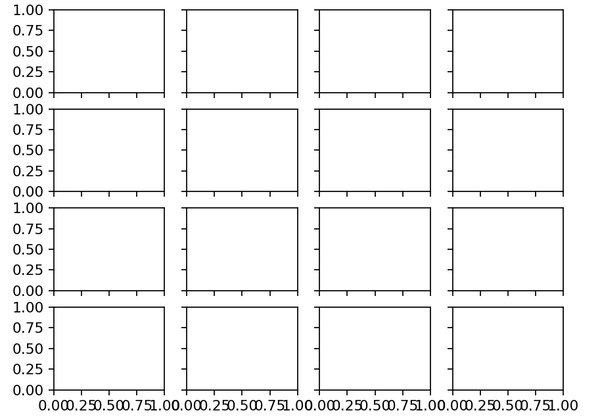# 自定义坐标轴刻度

Matplotlib图形对象具有层级关系。Figure对象其实就是一个盛放图形元素的盒子box，每个figure都会包含一个或多个axes对象，而每个axes对象又会包含其它表示图形内容的对象，比如xais和yaxis，也就是x轴和y轴。

• 主要刻度和次要刻度

```ax = plt.axes(xscale='log', yscale='log')
``````>>> ax.xaxis.get_major_locator()
<matplotlib.ticker.LogLocator at 0x276ff49db38>
>>> ax.xaxis.get_major_formatter()
<matplotlib.ticker.LogFormatterSciNotation at 0x276ff49d978>

>>> ax.xaxis.get_minor_locator()
<matplotlib.ticker.LogLocator at 0x276ff49d940>
>>> ax.xaxis.get_minor_formatter()
<matplotlib.ticker.LogFormatterSciNotation at 0x276ff493320>
```

• 隐藏刻度与标签

```ax = plt.axes()
x = np.linspace(0,10,100)
ax.plot(np.sin(x))

ax.yaxis.set_major_locator(plt.NullLocator())
ax.xaxis.set_major_formatter(plt.NullFormatter())
```• 设置刻度数量

```fig, ax = plt.subplots(4, 4, sharex=True, sharey=True)
for axi in ax.flat:
axi.xaxis.set_major_locator(plt.MaxNLocator(4))
axi.yaxis.set_major_locator(plt.MaxNLocator(4))
```